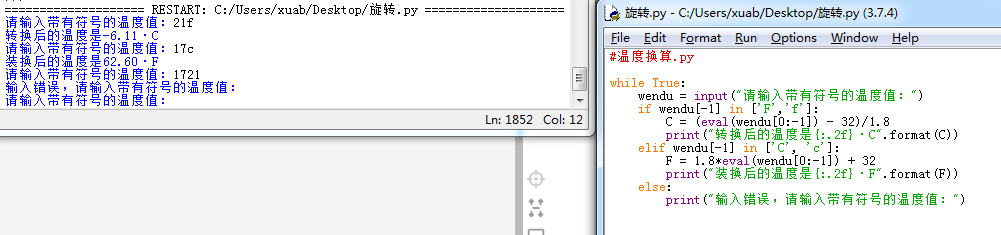• 主要介绍了C#华氏温度和摄氏温度相互转换的方法,涉及C#数学运算的相关技巧,非常简单实用,需要的朋友可以参考下
• 利用python实现简单的华氏温度和摄氏温度转换，采用python实现。
• labview 温度单位换算 摄氏度华氏度℉ 开尔文K,大家试试
• 华氏度转换二氧化硅matlab代码的MATLAB 这是要练习的四个MATLAB问题。 问题概述： 问题1） Define the functions x and y in terms of parameter a: x（t）=√tsin（t）y（t）=√tcos（t） Create a separate figure...
• 例如，将华氏温度转换摄氏温度。 temp_far = 100; temp_cel = far2cel(temp_far); 显示（temp_cel） 还包括一个名为 tempdim 的函数。 这与 MATLAB 的 distdim 非常相似。 它接收温度、它的单位以及您想要将其...matlab
• #温度换算.py while True: wendu = input(“请输入带有符号的温度值：”) if wendu[-1] in [‘F’,‘f’]: C = (eval(wendu[0:-1]) - 32)/1.8 print(“转换后的温度是{:.2f}·C”.format©) elif wendu[-1] in [‘C...

#温度换算.py
while True:
wendu = input(“请输入带有符号的温度值：”)
if wendu[-1] in [‘F’,‘f’]:
C = (eval(wendu[0:-1]) - 32)/1.8
print(“转换后的温度是{:.2f}·C”.format( C))
elif wendu[-1] in [‘C’, ‘c’]:
F = 1.8*eval(wendu[0:-1]) + 32
print(“装换后的温度是{:.2f}·F”.format(F))
else:
print(“输入错误，请输入带有符号的温度值：”)

运行结果如下图：展开全文python 入门
• 华氏度和摄氏度转换for-Python

千次阅读 2018-05-29 17:03:17
#python实现华氏度和摄氏度转换*获取用户输入的华氏度或者摄氏度自动转换**代码我个人觉得比较简短了*PS：刚学完的python，打算还是写一些代码存着，以便以后好查看def fc(): while True: fc=input("请输入...

#python实现华氏度和摄氏度转换

*获取用户输入的华氏度或者摄氏度自动转换*

*代码我个人觉得比较简短了*

PS：刚学完的python，打算还是写一些代码存着，以便以后好查看

def fc():
while True:
fc=input("请输入华氏度OR摄氏度，如：37.2C或者5.6F.输入'Q'或'q'退出\n")#获取用户输入的华氏度和摄氏度
if fc.endswith('C')==True:#判断输入的是否为摄氏度
c=float(fc.split('C'))#将输入的摄氏度使用split以C分割，将其分割成列表并取第一个值转换成浮点型。等同于 a=fc.split('C')   c=float(a)
print(fc,'的华氏度为:%fF' %(c*(9/5)+32))
elif fc.endswith('F')==True:#判断输入的是否为华氏度
f=float(fc.split('F'))
print(fc,'的摄氏度为:%fC' %((f-32)*5/9))
elif fc=='Q' or fc=='q':
break
elif fc.endswith('C')==False or fc.endswith('F')==False:
print('请输入正确的华氏度(F)或摄氏度(C),以F或C结尾')
fc()

展开全文Python
• print("求华氏度摄氏度之间的转换？") number = input("请输入带有单位的温度：") if number[-1] in ["f","F"]: #判断用户输入的最后一个字符是否为华氏度单位 C = (eval(number[0:-1]) - 32) / 1.8 print(...
print("求华氏度与摄氏度之间的转换？")

number = input("请输入带有单位的温度：")

if number[-1] in ["f","F"]:	#判断用户输入的最后一个字符是否为华氏度单位
C = (eval(number[0:-1]) - 32) / 1.8
print("转换后的温度是{:.2f}".format(C)+"C")
elif number[-1] in ["c","C"]:#判断用户输入的最后一个字符是否为摄氏度单位
F = eval(number[0:-1]) * 1.8 + 32
print("转换后的温度是{:.2f}".format(F)+"F")
else:#如果都不是，则输入有误
print("输入的温度有误！")

展开全文python
• 我们在去国外旅游的时候，经常会遇到一个小问题，我国的温度使用摄氏温度来度量，而一些国家喜欢使用华氏温度来度量，因此我们在国外看天气预报或者使用空调时会需要进行温度转换，同样，国外的游客来中国旅游时也会...
• 华氏度和摄氏度的换算

千次阅读 2021-01-11 23:29:20
扩展数据水的冰点是0摄氏度(0℃)，是32华氏度(32℉)，也就是说，用摄氏温度表测量，水到0°结冰；如果用华氏温度测量，水的结冰点是32°温度是一种物理量，代表物体的热度冷度。从微观意义上讲，它是用于测量...

F = 32 + C×1.8

C =

(华氏

-32

)÷1.8

325℉= 162.78℃300℉= 148.89℃

华氏量表是经验量表之一。

在美国的日常生活中，

经常使用这种

温度标度，并带有字母“

F”。

扩展数据

水的冰点是

0

摄氏度

(0℃)

32

华氏度

(32℉)

也就是说，

用摄氏温度表测量，

水到

0°结冰；

如果用华氏温度测量，

水的结冰点

32°

温度是一种物理量，代表物体的热度和冷度。从微观意义上讲，

它是用于测量分子热运动强度的物理量。

温度只能通过物体随温度变

化的某些特性间接测量。

用于测量物体温度的标度称为温度标度。

指定温度读数的起点(零点)和测量温度的基本单位。

世界上最常用的温度标度是华氏度标(°

f

)

，摄氏度(°C)

，热力

学温度标度(

k

)

，里氏温度标度(°Ré)

，兰金温度标度(

R

)和国际

实用温度规模。

从分子动力学理论的角度来看，

温度是分子平均动能

的标志。温度是大量分子热运动的集体表达，具有统计意义。但是对

于单个分子，温度没有意义。

摄氏温度已被纳入国际单位制。

在物理学中，

摄氏温度标度表示

t

，绝对温度标度(单位：开尔文)表示为

t

，转换关系为

t

=

t-

273.15

。摄氏温度是摄氏

1 K = 1℃时代的开尔文的特殊名称。

包括中国在内的世界上绝大多数国家使用摄氏度。

世界上只有五

展开全文• TempStr=input("请输入带有符号的温度值，C/c表示摄氏度、F/f表示华氏温度：") if TempStr[-1] in ['F','f']: C=(eval(TempStr[0:-1])-32)/1.8 print("转换后的温度是{:.2f}C".format(C)) elif TempStr[-1] in ['C...python 编程语言
•temp
• 用matlab语言编写的计算摄氏度华氏度之间的转换，可选择转换摄氏度还是华氏度。m文件，需要用matlab打开。matlab
• 华氏度摄氏度的转化（c语言） List item #include<stdio.h> int main() { int f; float c; for (f = 0; f <= 300; f = f + 10) { c = 5.0 / 9.0*(f - 32); printf("%4d\t %6.1f\n", f, c); } ...c语言
• package shangJiPractice; import java.util.Scanner; public class TemperatureConversion { ... System.out.print("请输入一个华氏温度："); double fah = sc.nextDouble(); System.out.pjava 算法
• /*将摄氏温度转为华氏度，直接乘以9，除以5，再加上32即可 从华氏度变成摄氏度你只要减去32，乘以5再除以9就行了.*/ int hd=0; //华氏温度 int sd=0; //摄氏温度 int i=0; System.out.println("欢迎使用本...java
• 题目：用python编写程序，输入华氏摄氏度，按公式c=5/9(f-12)计算输出摄氏温度，结果保留2位小数，输出格式为"c=%.1f\n"。 f = int(input()) print("c=%.1f\n"%(5/9*(f-12))) 例如： 输入温度：35 输出结果：...python 机器学习 自然语言处理
• 输入华氏温度，输出结果为摄氏温度，matlab函数，添加后使用matlab
• } } 扩展资料： 注意事项 /* * 华氏温度和摄氏温度互32313133353236313431303231363533e59b9ee7ad9431333431373331相转换，从华氏度变成 摄氏度你只要减去32，乘以5再除以9就行了，将 摄氏度转成华氏度，直接乘以9...
• 一开始看慕课上的小白课程就自己尝试写了一下华氏温度和摄氏温度的代码，然后发现报错，我又自己开始找问题，最后发现跟老师代码一样还是报错，小白就很无奈 然后慢慢查。。。最后得到了一份满意的代码： tem=...python
• 使用 C++ 语言，实现下面这段 python 代码所实现的 温度转换 功能， 体会 python 语言的简洁高效。 C++ 代码实现温度转换 #include <iostream> #include <string> #include <cstdio> using ...
• public static void main (String[]args){ Scaner input = new Scaner(System.in); double f = input.nextDouble(); ... System.out.println("华氏度:"+f) System.out.println("摄氏度:"+c) }java
• import java.util.Scanner; class T4 { public static void main(String[] args) { Scanner in=new Scanner(System.in); int a=0;//接收选择的 float x;//存温度的 System.out.println("请...华氏温度--->摄氏温...
• C语言 摄氏度与华氏度转换

千次阅读 2021-03-27 01:09:32
华氏度摄氏度公式：C=5/9*(F-32) //华氏度摄氏度公式：C=5/9*(F-32) #include<stdio.h> void main(){ double F,C; printf("%请输入华氏度："); scanf("%lf",&F); C=5.0/9*(F-32); printf("摄氏...c语言
• 温度的刻画有两个不同体系：摄氏度（Celsius）和华氏度（Fahrenheit）。‪‬‪‬‪‬‪‬‪‬‮‬‫‬‫‬‪‬‪‬‪‬‪‬‪‬‮‬‪‬‮‬‪‬‪‬‪‬‪‬‪‬‮‬‪‬‪‬‪‬‪‬‪‬‪‬‪‬‮‬‭‬‫‬‪‬...python
• 利用C语言怎么将摄氏度转换华氏度发布时间：2021-01-13 16:52:32来源：亿速云阅读：346作者：Leah这期内容当中小编将会给大家带来有关利用C语言怎么将摄氏度装换为华氏度，文章内容丰富且以专业的角度为大家分析...
• 华氏温度和摄氏温度转换-C语言 #include<stdio.h> int main() { double f, c; scanf_s("%lf", &f); printf("%f", 5 * (f - 32) / 9); return 0; }c语言
•eclipse...